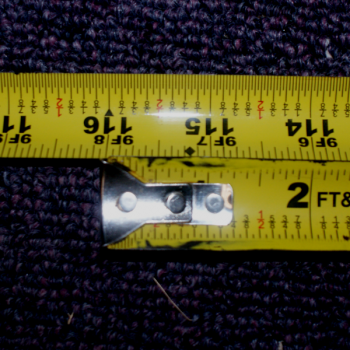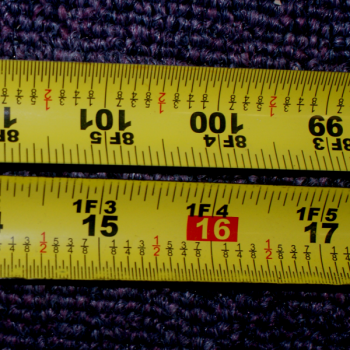Games
Problems
Go Pro!

Open up a flexible tape measure, until the year of your birth (last two digits) plus 100 is showing. Now bend the tape measure over and bring back the end of it until it lines up with your birth year. Now find the last two digits of the current year, and see what corresponds to it on the other side of the loop you've created. Take the last two digits of that number and subtract it from 100 and you'll have your age. Why does this work?

First of all, let's start by setting some limitations on this, because it doesn't always work. A couple towns over from us there is a woman who just turned 106. If we try this trick for her age, we find out that she's 6 years old. So if someone is over a hundred, we have to use a little common sense and say, "Well, she doesn't look like she's 6 years old, so we'll add 100 to that."

So in exploring this, we'll ignore any age 100 or more.

Also, we need to agree that we're talking about "age at the end of this year," because if the person hasn't yet had their birthday, this trick is off by one year.With those things clarified, here's an example. My grandmother was born in 1916, so I'm going to fold over the tape measure to look like this.

If I scan back up the tape measure until I find the current year (2015), I find that the number across from it is 101. Now I take the last two digits (01) and subtract that from 100, getting 99 (which is my grandmother's age).Let's stop for a minute and think about what we've done. We have a total of 116 inches extended on the tape measure. What significance does that number have?

Let's define some variables and see if we can make some sense of how that number is going to play into the tape measure game.

Let C = the current year (last two digits).
Let Cf = the first two digits of the current year (for example, 20 for the year 2015).
Let A = my grandmother's age at the end of the current year.
Let B = my grandmother's birth year (last two digits).
Let Bf = the first two digits of my grandmother's birth year (19 for the year 1916).

Since the birth year is the current year minus the age,

(100Bf + B) = (100Cf + C) - A

Let's do a little algebraic manipulation on this equation, rearranging terms until C - B is by itself.

C - B = A - 100(Cf - Bf

On the left side of the tape, I see the number B + 100. On the right side, I see 0. Since the left side is increasing from top to bottom, and the right is decreasing from top to bottom, every time I move up a number on the right, I'm moving down a number on the left.

Thus, if I move up to C on the right, I'm decreasing the value on the left by C, giving me: B + 100 - C.

Let's take that B + 100 - C and rearrange it:

100 - (C - B)

Since we found C - B in a previous equation, we can substitute this:

= 100 - [A - 100(Cf - Bf)]

= 100(Cf - Bf + 1) - A

Since we're only looking at the last two digits of the number, we can do a bit of modulus (remainder) math*, and say that 100(Cf - Bf + 1)  is congruent to 100 (mod 100), and therefore, what we're looking at is:

100 - A.

What happens if you subtract that from 100?

= 100 - (100 - A)

= 100 - 100 + A

= A (Current age!)

And there you have it. That's the algebra behind why this trick works.

As an added bonus, the trick also works if you just slide the tape measure out to the birth year, instead of 100 more than the birth year. BUT now we have the stipulation that it doesn't work if we haven't extended enough to get the current year on the right-hand side of the tape measure. So we could say, "Slide the tape measure out until the current year is on the right, and then keep sliding it until the last two digits on the left match the birth year." This saves you having to go out an extra 100 inches in many cases.

* This step of doing some modulus math is the reason the trick doesn't work for people over the age of 100; what we're actually finding is their age mod 100, or the remainder when their age is divided by 100. This has the effect of returning only the last two digits of their age.# Blogs on This SiteReviews and book lists - books we love!The site administrator fields questions from visitors.Like us on Facebook to get updates about new resources